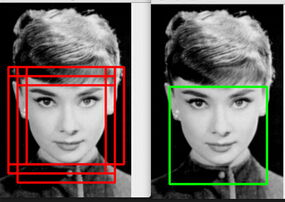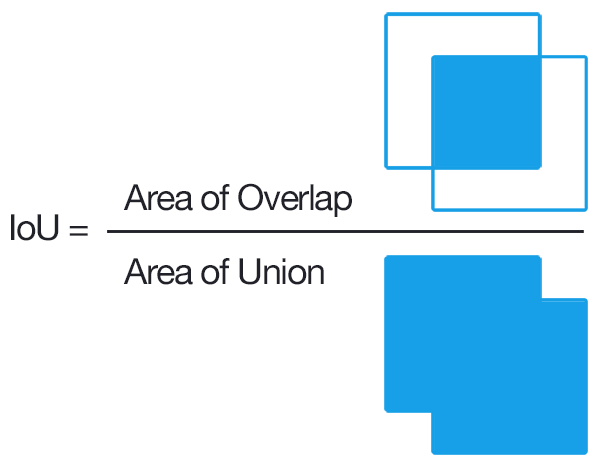시간 제한메모리 제한제출정답맞힌 사람정답 비율
20 초 (추가 시간 없음) 256 MB111100.000%

## 문제

Non-maximum suppression (NMS) has been widely used in several aspects of computer vision and is an integral part of many object detection algorithms. One of the most common problems in object detection is that an object might be detected multiple times. NMS technique ensures we get only a single detection per object.For each class, a list of bounding boxes is proposed by the algorithm. Each box is associated with a score, indicating the confidence the algorithm thinks there is an object of the given class inside the box. Now let's see how the NMS procedure works. In the beginning, all the boxes are unselected and non-suppressed.

1. First, select the box with the highest confidence score among boxes that are unselected and non-suppressed.
2. Then, look at all the unselected boxes in the image. If the IoU of an unselected box and a selected box is strictly larger than a given threshold, then the unselected box will be suppressed (discarded). We measure how two boxes are overlapped by the concept of Intersection over Union (IoU).1. Repeat the above procedure until all non-suppressed boxes are selected.

In this problem, we assume there is only one class, and simplify the object detection algorithm by only proposing square shaped boxes of the same size. Given the IoU threshold and a list of congruent square boxes proposed by the algorithm, can you report all selected boxes after going through the NMS procedure?

## 입력

The first line of the input gives the number of test cases, $T$ ($1 \le T \le 10$). $T$ test cases follow.

For each test case, the first line contains two integers $n$, $S$ ($1 \le n \le 10^5$, $1 \le S \le 10^7$) and a floating-point number $threshold$ ($0.300 \le threshold \le 0.700$), indicating the number of square bounding boxes proposed by the detection algorithm, the size of the squares, and the IoU threshold. The $threshold$ is exact and is given to the third digit after the decimal point.

In the next $n$ lines, each line contains two integers $x$, $y$ ($0 \le x < x + S \le 10^7$, $0 \le y < y + S \le 10^7$) --- the coordinates of the bottom left corner of a square box, followed by a floating point number $score$ ($0.0 \le score \le 1.0$) --- the score of this box. Scores are exact and have at most six digits after the decimal point. Also, scores are distinct.

## 출력

For each test case, the output starts with a line containing "Case #x: y", where x is the test case number (starting from 1), and y is the number of selected bounding boxes after going through the NMS procedure. The next line contains y integers indicating the indices of the boxes (starting from 1) in ascending order.

## 예제 입력 1

1
3 4 0.390
0 0 0.9
1 1 0.8
2 2 0.7


## 예제 출력 1

Case #1: 2
1 3


## 노트

There are $3$ boxes in the example: $[0, 4] \times [0, 4]$, $[1, 5] \times [1, 5]$ and $[2, 6] \times [2, 6]$, which are already in descending order of score. The second box is suppressed because the IoU of the first and the second box is $\frac{9}{23}$, which is strictly larger than $0.390$, the IoU threshold. The third box is selected because the IoU of the first and the third box is $\frac{1}{7}$, which is smaller than the IoU threshold.